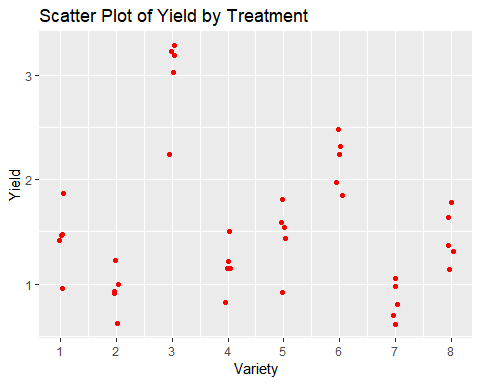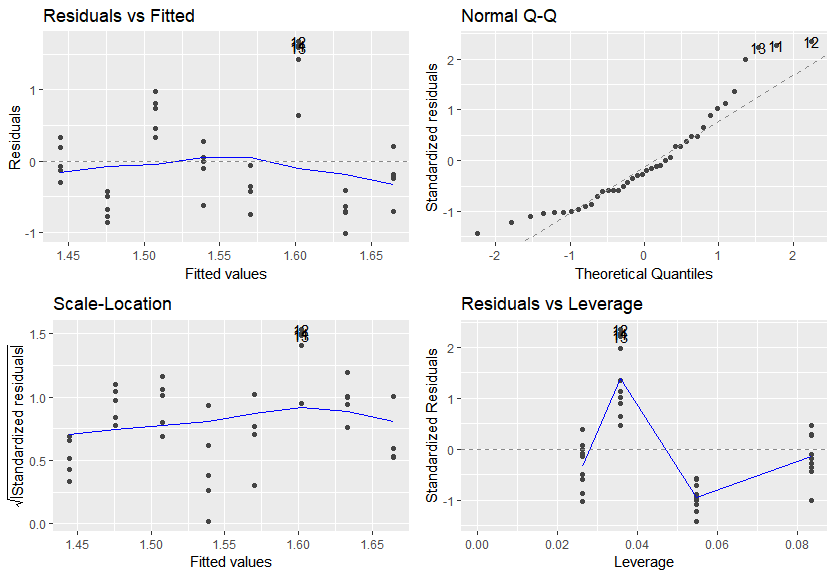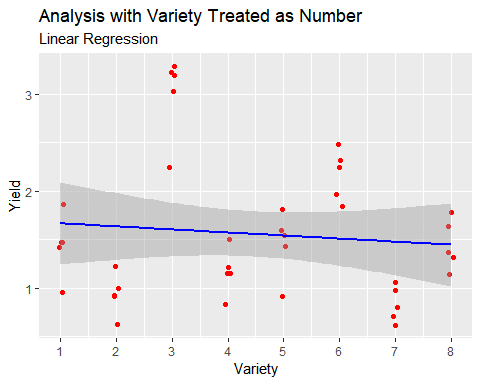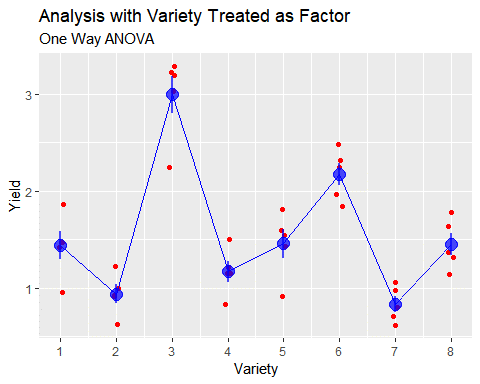# Blog

Statistics for Sustainable Development > Blog > A Problem with a Number of Factors

Technical Pieces

## A Problem with a Number of Factors

### Introduction

A large part of my job involves helping researchers who are working on analysing their own data, by checking over their work, helping with interpretations of results, and making suggestions for additional pieces of analysis. Through this, there are a number of common mistakes I see; some major and some minor. Usually when coming across these, I can explain the problem and refer the researchers to relevant journal articles or websites, so they can learn more. However, there’s one particular mistake I have come across more times than I can count, and yet when asked to give a reference for it, I have really struggled to find anything particularly coherent, outside of a few good responses on StackOverflow.  We even recently included an example of this mistake as part of an assessment when recruiting for a new member of our team, to see if they could identify it.

I think it falls into something of a grey area where it is too obvious and trivial for statisticians to directly address, and yet not in any way obvious to non-statisticians trying to battle away at an analysis of their own data.

So, purely so that I can reference myself in the future when this comes up (as I am at least 95% sure that it will!), let me explain.

### The Context

The set up is simple: I have some data from an agricultural experiment, where I have measured yield of crops across some different varieties. In this case, I’ve got 8 different varieties of my crop, and I have used a big field with 40 plots where I have randomly allocated 5 plots to be planted with each of the varieties. I would like to do some analysis to find out which of my varieties are getting high yields, and whether I can conclude that there are statistically significant differences in the average yield between my varieties.

I am a good researcher, so before doing any further analysis I explore my data a bit to see the results:Variety Mean Yield (t/ha) SD Yield (t/ha) 1 1.44 0.32 2 0.94 0.22 3 2.99 0.43 4 1.17 0.24 5 1.46 0.33 6 2.17 0.26 7 0.83 0.18 8 1.45 0.25

So from my initial exploration of the data, it looks like I might be on to a winner! Variety 3 looks like a consistently high yielding variety compared to the others. Time to complete my analysis with a simple one-way Analysis of Variance, get the surely inevitable tiny p-value indicating statistical significance, and then bask in the glory of my exciting and important new scientific finding.

` ## Analysis of Variance Table ##  ## Response: Yield ##           Df Sum Sq Mean Sq F value Pr(>F) ## Variety    1  0.208 0.20800  0.3925 0.5347 ## Residuals 38 20.138 0.52995`
` `

Wait, what? How is it that I can be getting a p-value of only 0.535, indicating no evidence of any treatment effect, when I look at the clear separation between the varieties that I can see in my graph, and the large differences in the means?

### The Diagnosis

At no point in any of the above analysis did my software give me any errors, or any explicit indication that what I was doing was incorrect. But what I did was indeed fundamentally incorrect. This issue is also not software dependent. Some of you may have recognised the output as coming from R; but variations on this same problem will occur whether you are using SAS, SPSS, Minitab, Genstat, Stata and probably any other software package you can think of.

The more eagle-eyed of you may have spotted the problem already. The only clue that something has gone wrong is in the df (degrees of freedom) column of the ANOVA table. Cast your mind back to the Stats 101 course where you may have first learned about ANOVA, or frantically check the relevant Wikipedia page (https://en.wikipedia.org/wiki/One-way_analysis_of_variance). Doing so will tell you that the number of degrees of freedom for our variety term should be J-1, where J is the total number of varieties. So in this example J is equal to 8, and we would therefore expect to have 8-1=7 degrees of freedom for our Variety term. But in the output, we only had 1 degree of freedom - so this is a way of diagnosing that not everything in our model is as we wanted it to be.

Often the advice for diagnosing model errors, as I would have been taught in my Stats 101 class, would have been to review some of the standard model checking plots. In general, this is good advice, but checking these here would not really have shed any light on the problem if we had already gotten this far without working out what the problem was (or indeed that we had a problem at all).There are no real issues with the homogeneity or normality or extreme outliers. The problem can be seen in these plots, from the residuals vs fitted plot, but it is a fairly subtle one to identify.

### The Problem

Variety is a coded variable, where the numbers 1 to 8 each represent a code for a different variety. My software package does not know this - it sees the numbers 1 to 8 and assumes that these are numbers, not codes. Therefore, rather than looking at whether the means across my 8 varieties are different to each other (ANOVA), my analysis is in fact looking at whether there is a trend in the yield as we increase the variety number (Linear Regression).

So, with variety treated as a number, the model I am fitting on my data looks like this:My 8 varieties are coded as 1 to 8 simply as a matter of convenience. There is no increasing sequence across my 8 varieties. Often when I have encountered this problem “in the wild”, then the order of 1 to 8 will simply reflect the alphabetical order of the variety names. Therefore analysing my data as if these codes were a set of numbers would be fundamentally incorrect, and any p-values or conclusions drawn from this analysis would be total irrelevant nonsense, as is the trend line being drawn onto that graph.

What I need is a model which can be fitted onto my data as below, considering all 8 varieties separately.The reason why this can happen so easily is that linear regression models, ANOVA, ANCOVA, even t-tests, are all in fact the same thing. There is a well written blog post explaining this here: https://www.theanalysisfactor.com/why-anova-and-linear-regression-are-the-same-analysis/. Most statisticians realise this, so generally want to make general purpose statistical tools rather than separating all these slightly different applications into completely different boxes. In R, but also in many other widely used software packages, the same functions for analysis can be used for any general linear model, and the underlying properties of the data determines how each variable will be treated rather than the use of a different function. Most non-statisticians don’t realise this, as they were usually taught about all of these methods in isolation, and are often taught with “perfect” example datasets where this sort of real-world issue would not be addressed. So it can be very easy to assume that where following a set of instructions of doing a One-Way ANOVA, that this will work on their own data, without considering the different encoding of their variables.

This problem would not have arisen if my varieties had been coded as A, B, C, D, E, F, G and H, or had been coded with their full names.

There are indeed occasions where we would want to treat our factor variables as numbers. For example, if we had applied 8 quantities of fertiliser to the plots, instead of 8 different varieties. In this instance, we might be interested in both the overall ANOVA, to see if there were significant differences between our 8 treatments, but also the regression, to better quantify the expected yield gain from adding an extra 1 kg of fertiliser.

### The Solution

Depending on your software package of choice, then convert your categorical variables into factors, or correctly specify which variables are factors in the model. This is easy in every half-decent software package. Many solutions to this problem will start talking about the need to calculate ‘dummy indicator variables’. As long as you are using a half-decent software package, this is not something that you have had to do yourself for over 20 years, so I would suggest largely ignoring anyone making that suggestion.

• In R you can convert variables to factors using as.factor()
• In SAS you would use a class statement within the relevant PROC
• In Genstat you can easily convert to factors through the data window, or when working through the import data routines
• In Stata you need to use the xi: functionality of the relevant function
• In SPSS you need to make sure the factor variables are included as factors rather than covariates.

Successfully doing so will give you something a lot more sensible, and in inline with what was expected:

` ## Analysis of Variance Table ##  ## Response: Yield ##           Df  Sum Sq Mean Sq F value    Pr(>F)     ## Variety    7 17.6713 2.52447    30.2 2.283e-12 *** ## Residuals 32  2.6749 0.08359                       ## --- ## Signif. codes:  0 '***' 0.001 '**' 0.01 '*' 0.05 '.' 0.1 ' ' 1`
` `

Finally! Now I can bask in the glory of my tiny p-value, and celebrate my surely inevitable Nobel prize that must be incoming for my wondrous discovery :)

### The Conclusion

There is no problem if you have a dataset with categorical/factor/nominal (all of these mean the same thing) variables coded as numbers. There is a problem if you analyse the data as if these were numbers - the results from any form of statistical model will be completely incorrect. Hopefully, if you have made it this far you might have noticed that the title of this blog is not really correct – it is in fact not “a problem with a number of factors” but rather “a problem with a factor of numbers”!##### Author: Sam Dumble

Sam has been part of SSD since its inception in 2016. Given the importance and frequent misinterpretation of statistics, he is keen to ensure that results are presented in a clear and understandable manner, particularly through the use of graphics and maps. He does most of his analysis using the software packages R and QGIS. He is also an experienced user of various other packages such as SAS, SPSS, Minitab, Genstat, Stata and CSPro.